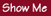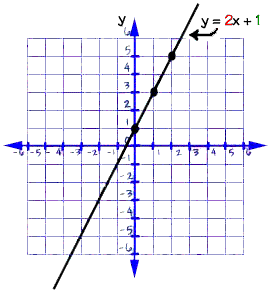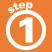Home    |    Teacher    |    Parents    |    Glossary    |    About UsEvery straight line can be represented by an equation: y = mx + b. The coordinates of every point on the line will solve the equation if you substitute them in the equation for x and y.

The slope m of this line - its steepness, or slant - can be calculated like this:

m = change in y-value
change in x-value

The equation of any straight line, called a linear equation, can be written as: y = mx + b, where m is the slope of the line and b is the y-intercept.The y-intercept of this line is the value of y at the point where the line crosses the y axis.Homework Help | Algebra | Graphing Equations and InequalitiesEmail this page to a friendSearch·  The coordinate plane·  Slope and y-intercept·  Graphing linear     equationsFirst Glance In Depth Examples WorkoutSlope and y-intercept